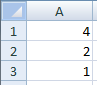# Evaluation model

The evaluation model of the Power Query M formula language is modeled after the evaluation model commonly found in spreadsheets, where the order of calculations can be determined based on dependencies between the formulas in the cells.

If you have written formulas in a spreadsheet such as Excel, you may recognize the formulas on the left will result in the values on the right when calculated:In M, an expression can reference previous expressions by name, and the evaluation process will automatically determine the order in which referenced expressions are calculated.

Let's use a record to produce an expression which is equivalent to the above spreadsheet example. When initializing the value of a field, you refer to other fields within the record by the name of the field, as follows:

``````[
A1 = A2 * 2,
A2 = A3 + 1,
A3 = 1
]
``````

The above expression evaluates to the following record:

``````[
A1 = 4,
A2 = 2,
A3 = 1
]
``````

Records can be contained within, or nested, within other records. You can use the lookup operator ([ ]) to access the fields of a record by name. For example, the following record has a field named Sales containing a record, and a field named Total that accesses the FirstHalf and SecondHalf fields of the Sales record:

``````[
Sales = [ FirstHalf = 1000, SecondHalf = 1100 ],
Total = Sales[FirstHalf] + Sales[SecondHalf]
]
``````

The above expression evaluates to the following record:

``````[
Sales = [ FirstHalf = 1000, SecondHalf = 1100 ],
Total = 2100
]
``````

You use the positional index operator ({ }) to access an item in a list by its numeric index. The values within a list are referred to using a zero-based index from the beginning of the list. For example, the indexes 0 and 1 are used to reference the first and second items in the list below:

``````[
Sales =
{
[
Year = 2007,
FirstHalf = 1000,
SecondHalf = 1100,
Total = FirstHalf + SecondHalf // equals 2100
],
[
Year = 2008,
FirstHalf = 1200,
SecondHalf = 1300,
Total = FirstHalf + SecondHalf // equals 2500
]
},
#"Total Sales" = Sales{0}[Total] + Sales{1}[Total] // equals 4600
]
``````

## Lazy and eager evaluation

List, Record, and Table member expressions, as well as let expressions (Go to Expressions, values, and let expression), are evaluated using lazy evaluation. That is, they are evaluated when needed. All other expressions are evaluated using eager evaluation. That is, they are evaluated immediately when encountered during the evaluation process. A good way to think about this is to remember that evaluating a list or record expression will return a list or record value that knows how its list items or record fields need to computed, when requested (by lookup or index operators).